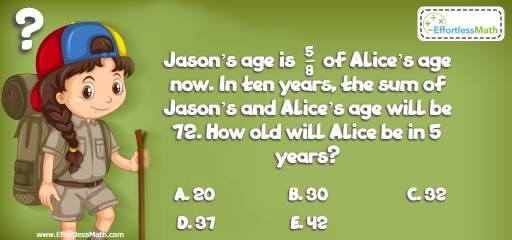# Algebra Puzzle – Challenge 55

Who can solve this great math puzzle? This is for smart people who love teasing their brains with math challenges!## Challenge:

Jason’s age is  $$\frac{5}{8}$$ of Alice’s age now. In ten years, the sum of Jason’s and Alice’s age will be 72. How old will Alice be in 5 years?

A- 20

B- 30

C- 32

D- 37

E- 42

### The Absolute Best Book to Challenge Your Smart Student!

Let J be the Jason’s age and A be the Alice’s age. Then:
J =$$\frac{5}{8}$$ A
In 10 years: (J + 10) + (A + 10) = 72
Replace J with its value in the first equation:
Then: $$(\frac{5}{8} A + 10) + (A + 10) = 72 →1 \frac{5}{8} A = 52 → A = 32$$
In 5 years, Alice will be 37. (32 + 5 = 37)

The Absolute Best Books to Ace Algebra

### What people say about "Algebra Puzzle – Challenge 55 - Effortless Math: We Help Students Learn to LOVE Mathematics"?

No one replied yet.

X
45% OFF

Limited time only!

Save Over 45%

SAVE $40 It was$89.99 now it is \$49.99